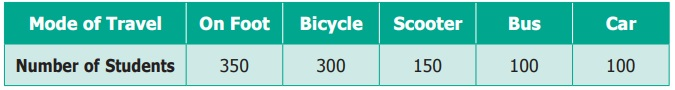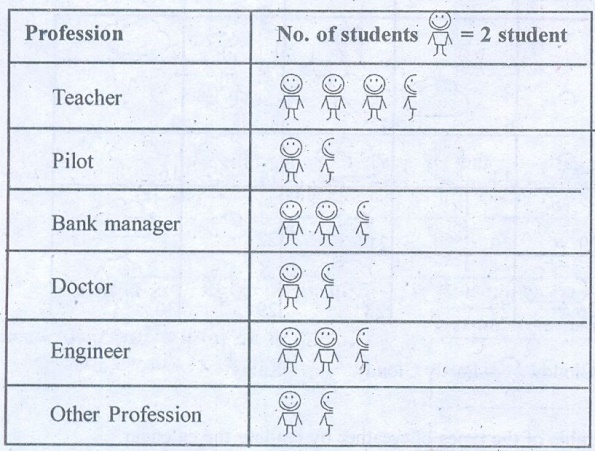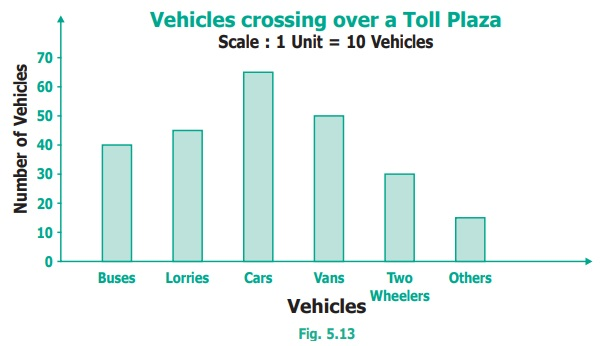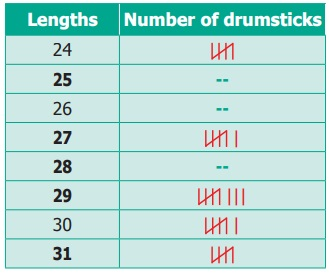Home | | Maths 6th Std | Exercise 5.4

# Exercise 5.4

6th Maths : Term 1 Unit 5 : Statistics : Exercise 5.4 : Miscellaneous practice problems, Challenging Problems : Text Book Back Exercises Questions with Answers, Solution

Exercise 5.4

Miscellaneous practice problems

1. The heights (in centimeters) of 40 children are.

110 112 112 116 119 111 113 115 118 120

110 113 114 111 114 113 110 120 118 115

112 110 116 111 115 120 113 111 113 120

115 111 116 112 110 111 120 111 120 111

Prepare a tally mark table.

Solution:2. There are 1000 students in a school. Data regarding the mode of transport of the students is given below. Draw a pictograph to represent the data.Solution:3. The following pictograph shows the total savings of a group of friends in a year. Each picture represents a saving of Rs.100. Answer the following questions.(i) What is the ratio of Ruby’s saving to that of Thasnim’s? Answer: 5 : 4

(ii) What is the ratio of Kuzhali’s savings to that of others? Answer: 5 : 9

(iii) How much is Iniya’s savings? Answer: Rs.300

(iv) Find the total amount of savings of all the friends? Answer: Rs.2400

(v) Ruby and Kuzhali save the same amount. Say True or False. Answer: True

Challenging Problems

4. The table shows the number of moons that orbit each of the planets in our solar system.Make a Bar graph for the above data.

Solution:5. The prediction of Weather in the month of September is given below.(i) Make a frequency table of the types of weather by reading the calendar.

Weather : No. of days

Sunny : 10

Partly cloudy : 6

Cloudy : 8

Rainy : 6

Total : 30

(ii) How many days are either cloudy or partly cloudy?

Answer: 6 + 8 = 14 days

(iii) How many days do not have rain? Give two ways to find the answer?

24 days

(i) Subtract Rainy days from total

(ii) Add sunny, partly cloudy and cloudy days

(iv) Find the ratio of the number of Sunny days to Rainy days.

6. 30 students were interviewed to find out what they want to become in future. Their responses are given in the following table.Represent this data using Pictograph.

Solution:7. Yasmin of class VI was given a task to count the number of books which are biographies, in her school library. The information collected by her is represented as follows.Observe the pictograph and answer the following questions.

(i) Which title has the maximum number of biographies?

(ii) Which title has the minimum number of biographies?

(iii) Which title has exactly half the number of biographies as Novelists?

(iv) How many biographies are there on the title of Sportspersons?

(v) What is the total number of biographies in the library?

8. The bar graph illustrates the results of a survey conducted on vehicles crossing over a Toll Plaza in one hour.Observe the bar graph carefully and fill up the following table.Solution:9. The lengths (in the nearest centimetre) of 30 drumsticks are given as follows.Draw the bar graph showing the same information.

Solution:Exercise 5.4

1)3) i) 5 : 4 ii) 5 : 19 iii) ₹ 300 iv) ₹ 2400 v) true

5) ii) 14 days iii) 24 days iv) 10 : 6

7) i) Novelists ii) Scientists iii) Sportspersons iv) 25 v) 160

Prasanta Chandra Mahalanobis(29.06.1893 – 28.06.1972)

He was born in Bikrampur in Bengal. He was the founder of Indian Statistical Institute and greatly helped in data analysis for Indian Government.

Tags : Questions with Answers, Solution | Statistics | Term 1 Chapter 5 | 6th Maths , 6th Maths : Term 1 Unit 5 : Statistics
Study Material, Lecturing Notes, Assignment, Reference, Wiki description explanation, brief detail
6th Maths : Term 1 Unit 5 : Statistics : Exercise 5.4 | Questions with Answers, Solution | Statistics | Term 1 Chapter 5 | 6th Maths